Open In App

# Partial Orders and Lattices (Set-2) | Mathematics

Prerequisite: Partial Orders and Lattices | Set-1

Well Ordered Set –
Given a poset, (X, ≤) we say that ≤ is a well-order (well-ordering) and that is well-ordered by ≤ if every nonempty subset of X has a least element. When X is non-empty, if we pick any two-element subset, {a, b}, of X, since the subset {a, b} must have a least element, we see that either a≤b or b≤a, i.e., every well-order is a total order.
E.g. – The set of natural number (N) is a well ordered.

Lattice: A POSET in which every pair of element has both a least upper bound and greatest lower bound.

### Types of Lattice:-

1. Bounded Lattice:
A lattice L is said to be bounded if it has the greatest element I and a least element 0.
E.g. – D18= {1, 2, 3, 6, 9, 18} is a bounded lattice.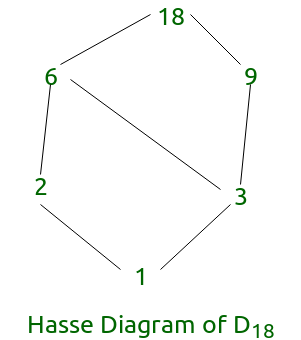Note: Every Finite lattice is always bounded.

2. Complemented Lattice:
A lattice L is said to be complemented if it is bounded and if every element in L has a complement. Here, each element should have at least one complement.
E.g. – D6 {1, 2, 3, 6} is a complemented lattice.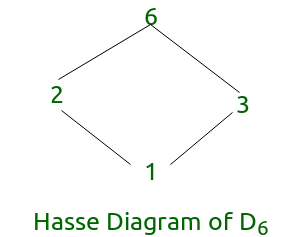In the above diagram every element has a complement.

Any element ‘b‘ will be the complement of an element ‘a‘ in the poset (X, ≤) which is lattice if and only if both condition below are satisfied -:

• glb(a, b) = least element
• lub(a,b) = greatest element

or when (a^b = least element and avb = greatest element) where,

greatest element (1) is element which is related by every other element in the lattice.

Mathematically, any element a, of poset (X, ≤) which is lattice , will be greatest element iff ∀ b∈X, (b,a)∈ R. where R is the relation on which element are related. Example in above diagram for D6, greatest element is 6 as it is related by every other element in the lattice i.e. (1,6), (2,6), (3,6) ∈ R.

least element (0) is element which relates to every other element in the lattice.

Mathematically, any element a, of poset (X, ≤) which is lattice , will be least element iff ∀ b∈X, (a,b)∈ R. where R is the relation on which element are related. Example in above diagram for D6, least element is 1 as it relates to every other element in the lattice i.e. (1,2), (1,3), (1,6) ∈ R.

Greatest and Least element in any set, if they exist will not be more than one unlike maximal and minimal elements which can be more than one.

3.Distributive Lattice:

If a lattice satisfies the following two distribute properties, it is called a distributive lattice.

• x ∧ (y ∨ z) = (x ∧ y) ∨ (x ∧ z)
• x ∨ (y ∧ z) = (x ∨ y) ∧ (x ∨ z)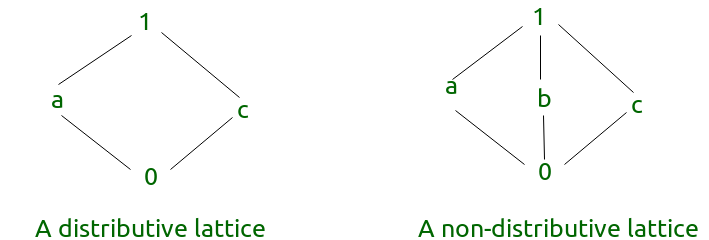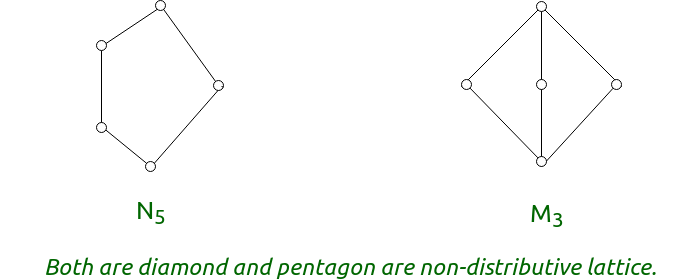• A complemented distributive lattice is a boolean algebra or boolean lattice.
• A lattice is distributive if and only if none of its sublattices is isomorphic to N5 or M3.
• For distributive lattice each element can have at most one complement. This can be used as a theorem to prove that a lattice is not distributive.

4.Modular Lattice

If a lattice satisfies the following property, it is called a modular lattice.
a^(b∨(a^d)) = (a^b)(a^d).

Example-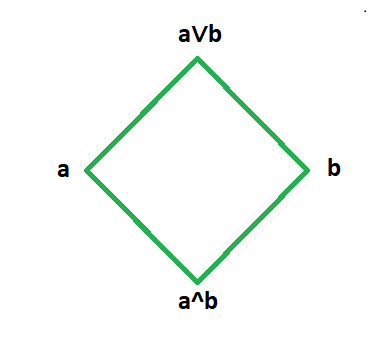5.Complete Lattice:-

A complete lattice is a special type of lattice where every subset of elements has a lower bound and an upper bound.

For example, Imagine a lattice based on the  ordering relation < including all natural numbers between 1 and 100.More detailed discussion on complete lattice is given below.

A lattice is complete if it has both a least and a greatest element. Is the statement correct?
Before discussing the correctness of this statement we need to know few mathematical definitions. We know that a lattice is a partially ordered set(poset ,that is, reflexive, antisymmetric and transitive) such that any pair of elements in the lattice  always has a greatest lower bound(glb) and a least upper bound(lub). Now greatest and least elements of a lattice are basically the glb and lub of all the lattice elements that is the element which is dominated by all the lattice elements and the element which dominates all the lattice elements.

As we have already explained the mathematical definitions. We now try to analyse our claim.

First Part:  If a lattice is complete then it has both a least and a greatest element.

Argument:  Consider a lattice D. Suppose, it is complete.  According to the definition of complete lattice, every subset of elements of D has a least upper bound (lub) and a greatest lower bound (glb).  It means, consider any arbitrary subset S of lattice D and S will have a lub and a glb.  Now, every set is considered as a subset to itself.

(Note that the definition clearly mentions subset and not proper subset)

So, D is a subset of itself.  It implies, subset D will have a lub (least element) and a glb (greatest element).  So, D  has  both  a  least  and  a  greatest  element.  Therefore, if a lattice is complete then it has both a least and a greatest element.

Second Part:  If a lattice has both a least and a greatest element then it is complete.

Counter Example:  Consider the lattice consisting of all the rational numbers in the interval [1,2]. This set has a least element as 1 and greatest element as 2.Now we take an irrational number in this interval say square root of 2. Now we try to create a subset of this lattice consisting of rational approximation of square root of 2.As square root of 2 being irrational will not belong to this set and hence the infinite number of approximations will not have a lub. The approximations can be written as follows:-

1.4<1.41<1.414<1.4142<1.41421<1.414213<1.4142135……………………….and it continues without any upper bound.

Now this set of rational approximations is an infinite but is indeed a subset without a least upper bound or rather an upper bound.

Similar argument can be given in case of glb which I leave to the readers as an exercise

Thus it is clear that a complete lattice will have both least and a greatest element but a lattice with both greatest and least element may not be complete.